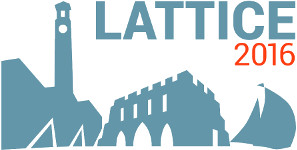#The 34th International Symposium on Lattice Field Theory (Lattice 2016)

Jul 24 – 30, 2016
Highfield Campus, University of Southampton
Europe/London timezone

## Charm physics by $N_f=2+1$ Iwasaki gauge and the six stout smeared $O(a)$-improved Wilson quark actions on a $96^4$ lattice

Jul 28, 2016, 2:00 PM
20m
Building B2a Room 2065 (Highfield Campus, University of Southampton)

### Speaker

Yusuke Namekawa (University of Tsukuba)

### Description

We present our results of charm physics in $N_f=2+1$ lattice QCD. Our calculation is performed on configurations generated with Iwasaki gauge and the six stout smeared $O(a)$-improved Wilson quark actions on a $96^4$ lattice at $\beta=1.82$ ($a^{-1} = 2.3$ GeV) with the spatial extent $L=8.1$ fm. The pion mass is almost physical $m_\pi=145$~MeV. The relativistic heavy quark action is utilized for the charm quark. We exhibit the charmed spectrum and the charm quark mass, focusing on stout smearing influence.

### Primary author

Yusuke Namekawa (University of Tsukuba)

 Slides